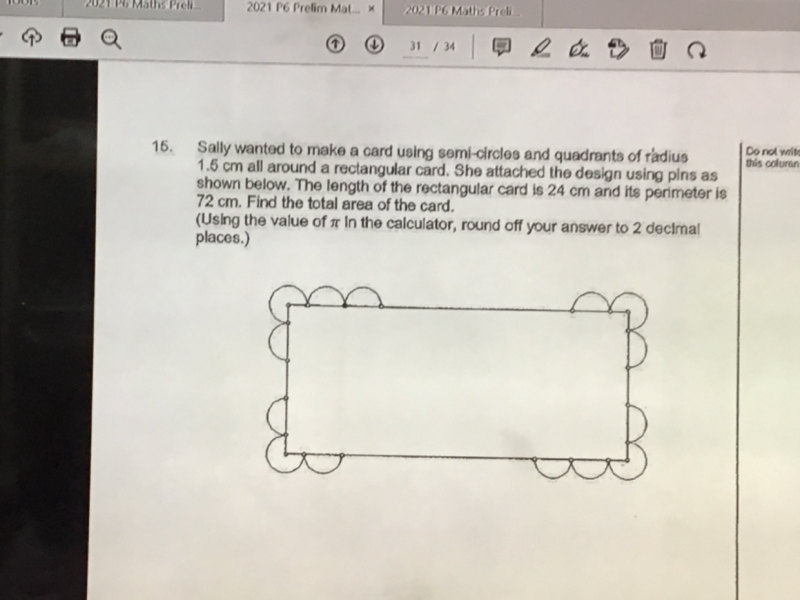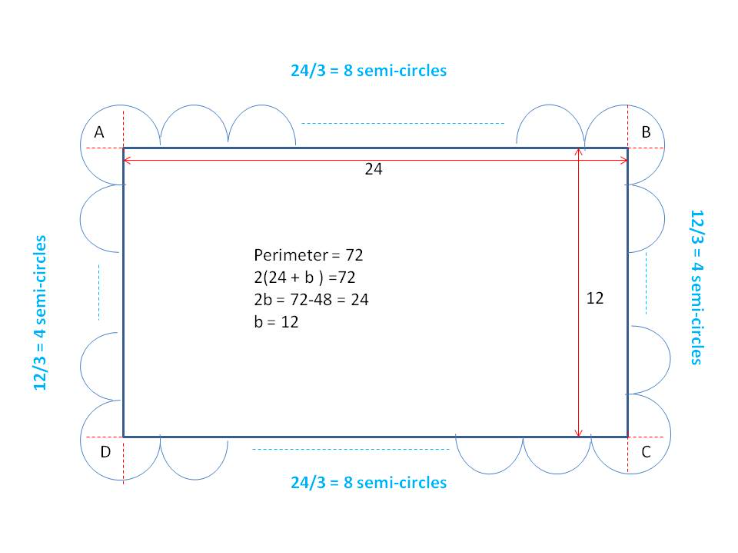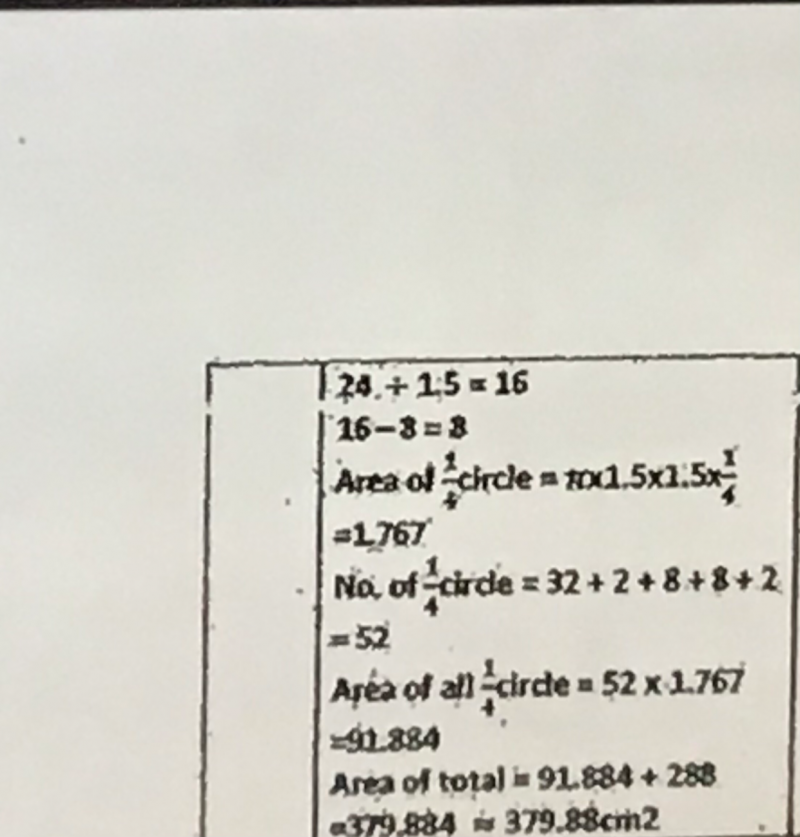# QuestionPls help to solve thxAh…sorry I read the question too quickly…it said “ALL AROUND” the rectangular card…. I just used the picture and started on working the area….hence missing out many more semi-circles…

Moral of the story, must read the entire question and not just look at the picture….I was tricked…lol

Perimeter = 72

2(24 + b ) =72

2b = 72-48 = 24   => b = 12

Area of rectangle = 12 x 24 = 288

Semi-circles along the length = (24/3) x 2 = 16

Semi-circles along the breadth = (12/3) x 2 =8

The A, B, C , D is made up of 2 semi-circles.

Hence total semi-circles = 16+8+2 = 26

Area of 26 semi-circles = [26 x π x (1.5) x (1.5)] / 2  = 91.89

Hence total area of card =  288 + 91.89 = 379.89cm2